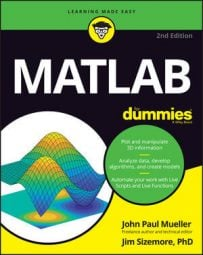##### MATLAB For DummiesYou need to know which operators MATLAB supports, but remember them all isn't easy. The following table provides a brief summary of the operators that MATLAB supports.

Operator Type Description Example
- Arithmetic Subtracts the right operand from the left operand. 5 - 2 = 3
* Arithmetic Multiplies the right operand by the left operand. 5 * 2 = 10
^ Arithmetic Calculates the exponential value of the right operand by the left operand. 5^2 = 25
/ Arithmetic Divides the left operand by the right operand. 5 / 2 = 2.5000
Arithmetic Divides the right operand by the left operand. 5 2 = 0.4000
+ Arithmetic Adds two values together. 5 + 2 = 7
. Arithmetic Modifies operators to perform element-by-element arithmetic vis-à-vis matrix arithmetic. You receive no modification if you're operating on scalars (ordinary numbers). [1,2]*[3;4] = 11

[1,2].*[3,4] = [3,8]
= Assignment Assigns the value found in the right operand to the left operand. MyVar = 2 results in MyVar containing 2
bitand Bitwise Performs a logical and of the bits in two numbers. bitand(4, 5) = 4
bitor Bitwise Performs a logical or of the bits in two numbers. bitor(4, 5) = 5
bitget Bitwise Obtains the value of the bit at a specific location. bitget(4, 3) = 1
bitset Bitwise Changes the bit at the specified location. bitset(4, 1, 1) = 5
bitshift Bitwise Shifts the bits the specified number of positions. bitshift(2, 1) = 4
bitxor Bitwise Performs a logical exclusive or on the bits in two numbers. bitxor(4, 5) = 1
and Logical Determines whether both operands are true. and(true, true) = 1 (or true)

and(true, false) = 0 (or false)

and(false, false) = 0

and(false, true) = 0
not Logical Negates the truth value of a single operand. A true value becomes false and a false value becomes true. not(true) = 0

not(false)=1
or Logical Determines when one of two operands are true. or(true, true) = 1

or(true, false) = 1

or(false, false) = 0

or(false, true) = 1
xor Logical Determines when one and only one of the operands is true. xor(true, true) = 0

xor(true, false) = 1

xor(false, false) = 0

xor(false, true) = 1
all Logical Determines whether all the array elements are nonzero or true. all([1, 2, 3, 4]) = 1

all([0, 1, 2, 3]) = 0
any Logical Determines whether any of the array elements are nonzero or true. any([0, 1, 0, 0]) = 1

any([0, 0, 0, 0]) = 0
~= Relational Determines whether two values are not equal. 1 ~= 2 is 1 (or true)
< Relational Verifies that the left operand value is less than the right operand value. 1 < 2 is 1
<= Relational Verifies that the left operand value is less than or equal to the right operand value. 1 <= 2 is 1
== Relational Determines whether two values are equal. Notice that the relational operator uses two equals signs. A mistake many developers make is using just one equals sign, which results in one value being assigned to another. 1 == 2 is 0
> Relational Verifies that the left operand value is greater than the right operand value. 1 > 2 is 0
>= Relational Verifies that the left operand value is greater than or equal to the right operand value. 1 >= 2 is 0
- Unary Negates the original value so that positive becomes negative and vice versa. -(-4) results in 4 while -4 results in -4
+ Unary Provided purely for the sake of completeness. This operator returns the same value that you provide as input. +4 results in a value of 4